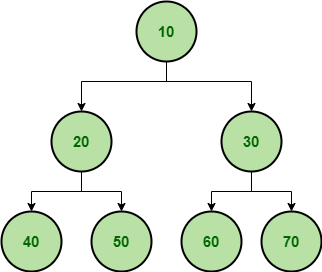GeeksforGeeks App
Open AppBrowser
Continue

## Related Articles

A binary tree is a tree that has at most two children for any of its nodes. There are several types of binary trees. To learn more about them please refer to the article on “Types of binary treeSample Binary Tree

Application of Binary Trees:

• Search algorithms: Binary search algorithms use the structure of binary trees to efficiently search for a specific element. The search can be performed in O(log n) time complexity, where n is the number of nodes in the tree.
• Sorting algorithms: Binary trees can be used to implement efficient sorting algorithms, such as binary search tree sort and heap sort.
• Database systems: Binary trees can be used to store data in a database system, with each node representing a record. This allows for efficient search operations and enables the database system to handle large amounts of data.
• File systems: Binary trees can be used to implement file systems, where each node represents a directory or file. This allows for efficient navigation and searching of the file system.
• Compression algorithms: Binary trees can be used to implement Huffman coding, a compression algorithm that assigns variable-length codes to characters based on their frequency of occurrence in the input data.
• Decision trees: Binary trees can be used to implement decision trees, a type of machine learning algorithm used for classification and regression analysis.
• Game AI: Binary trees can be used to implement game AI, where each node represents a possible move in the game. The AI algorithm can search the tree to find the best possible move.

Real-time applications of Binary Trees:

• DOM in HTML.
• File explorer.
• Used as the basic data structure in Microsoft Excel and spreadsheets.
• Editor tool: Microsoft Excel and spreadsheets.
• Evaluate an expression
• Routing Algorithms

• Efficient searching: Binary trees are particularly efficient when searching for a specific element, as each node has at most two child nodes, allowing for binary search algorithms to be used. This means that search operations can be performed in O(log n) time complexity.
• Ordered traversal: The structure of binary trees enables them to be traversed in a specific order, such as in-order, pre-order, and post-order. This allows for operations to be performed on the nodes in a specific order, such as printing the nodes in sorted order.
• Memory efficient: Compared to other tree structures, binary trees are relatively memory-efficient because they only require two child pointers per node. This means that they can be used to store large amounts of data in memory while still maintaining efficient search operations.
• Fast insertion and deletion: Binary trees can be used to perform insertions and deletions in O(log n) time complexity. This makes them a good choice for applications that require dynamic data structures, such as database systems.
• Easy to implement: Binary trees are relatively easy to implement and understand, making them a popular choice for a wide range of applications.
• Useful for sorting: Binary trees can be used to implement efficient sorting algorithms, such as heap sort and binary search tree sort.